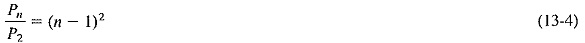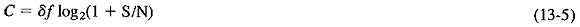## Noise in Information – Carrying Channel:

Noise in Information-carrying capacity of a channel. This idea will now be explored further, as will means of combating noise.

### Effects of Noise:

That Noise in Information has some harmful effect has already been demonstrated. To quantify the effect, consider again the earlier suggestion that each letter in the alphabet could be represented by a different signal amplitude, using 32-scale code. If this were done, the information flow would be greatly speeded (according to the Hart­ley law), since each letter would now be represented by one symbol instead of five. Unless transmitting power were raised tremendously, noise would cause so many er­rors as to make the multilevel system useless. The truth of this may be shown by considering the power required for the binary coding system and for any other system under the same noise conditions.

For a given transmission and coding system, there is such a thing as a threshold noise level; as long as noise does not exceed it, practically no errors occur. When a binary code is used, Noise in Information must compete with the full power of the transmitter to affect the signal, and practical results show that a signal-to-noise ratio of 30 dB ensures virtually error-free reception. This corresponds to a noise power of 1/1000 of signal power, i.e., an rms noise voltage of 1/31.6 of the rms signal voltage maximum. Let us take this S/N ratio as a practical requirement and consider the effect of this condition on increased signaling levels.

If it is now decided to double signaling speed by doubling the number of amplitude levels to four, the transmitted power will have to be increased to retain the 30-dB S/N ratio at the receiver. In terms of the maximum permitted amplitude, the new levels will be 0, 1/3, 2/3 and 1, where they were 0 and 1 in the binary system. This means that the difference in voltage levels is now one-third of what it was, the differ­ence in power levels is one-ninth, and therefore transmitted power must be multiplied ninefold when the signaling speed is doubled. Similarly, if an eight-level code is used, each amplitude level difference is one-seventh of the original, necessitating a 49-fold increase in transmitting power to return to the original 30-dB S/N ratio. Finally, if the proposed 32-level code were used, the power transmitted would have to be increased by a factor of 312 = 961. It is easy to deduce that this power increase is logarithmic and is given bywhere

n = number of levels in the code

Pn = power required in the n-level code

P2 = power level required in the binary code

In noise-limited conditions, the advantage of a binary system is such as to outweigh almost all other considerations.

### Capacity of a Noisy Channel:

The preceding section showed that transmitted power must be raised considerably, if a constant signal-to-noise ratio is to be kept when the number of coding levels is increased to raise the signaling speed. The Shannon-Hartley theorem gives a formula for the capacity of a channel when its bandwidth and noise level are known. This capacity iswhere

C = channel capacity, bits per second

δf = bandwidth, Hz

S/N = ratio of total signal power to total random noise power at the input to the receiver, within the frequency limits of this channel, i.e., over the bandwidth δf

The Shannon-Hartley theorem shows a limit that cannot be exceeded by the signaling speed in a channel in which the Noise in Information is purely random. It may be used as a very good approximation for the ultimate channel capacity of most transmission chan­nels, although practical noise distributions are never perfectly random. Example 13-1 shows the limiting channel speed for a typical telephone channel to be approximately 33 kilobits per second. Speeds used in practice over such channels do not normally exceed 10.8 kilobits per second (10.8 kbps). If the answer to Example 13-1 is equated with Equation (13-2), it will be seen that 39.8 code levels would be required to reach the Shannon speed limit for this channel, resulting in a system that is too complex in practice.

It would be incorrect to assume that doubling the bandwidth of a noise-limited channel will automatically double its capacity, that would be misinterpreting Equation (13-5). Consider the following example.

It is seen from the above example that capacity was increased, but certainly not doubled, when the bandwidth was doubled. This implies that useful possibilities of trading bandwidth for signal-to-noise ratio exist. Indeed, such tradeoffs are often made in system design, especially in power-limited situations. If channel capacity seems low in a given situation, this does not mean that a wanted amount of information cannot be sent over a given channel. As Equation (13-3) amply shows, it merely means that sending this amount of information takes longer.

Finally, it must be emphasized that the Shannon-Hartley theorem represents a fundamental limitation. The only consequence of trying to exceed the Shannon limit would be an unacceptable error rate. In practical transmission systems, error rates greater than 1 error in 105 are generally considered not good enough.

### Redundancy:

The preceding has assumed, although this was not stated explicitly at the time, that all messages send through the noise-limited channel were unpredictable. That is, they were assumed to be random, without any redundancy whatever. If redun­dant messages were sent, it is generally possible to work out from context the correct version of an erroneous message. Error rates can be very significantly reduced.

Redundancy is that which is not essential it can be removed from a signal and yet leave the remainder intelligible. All those who have sent telegrams which contain only the key words, leaving out all the articles and simple verbs, for instance, will have taken advantage of the redundancy in the language to save money. The letter “u” always follows the letter “q” in English, and so it is fully redundant.

Anyone with an ounce of imagination could work out the correct spelling of long words if they were transmitted with a couple of non-key letters missing. By sending a message over a noise-limited channel, from which most redundancy had been eliminated, it would be possible to increase the effective signaling speed quite substantially.

Scroll to Top